#### How to measure halo in MiniLED backlight?

Publisher: Supplier of LED Display Time: 2022-09-22 17:30 Views: 360

An important issue in MiniLED backlight display modules is how to optimize the halo effect, and equally important is how to measure and evaluate the halo, so as to know how much the optimization has improved the effect.

This paper introduces a method recently proposed by Samsung to measure the halo of MiniLED-backlit displays to quantify the variation of actual contrast with the viewing angle and length of the halo effect. Evaluation Index 1: Halo Length Before conducting the subjective evaluation experiment, try to quantify the halo length. Three MiniLED LCD displays are used: 17.3'' 240 partitions, 32'' 576 partitions, and 65'' 792 partitions. The D/I values (divisions/inch) of these 3 monitors are: 13.9, 18.0, and 12.2. As a supplement, both 15.6" and 65" self-luminous displays are used, and the number of pixels is considered to be the number of partitions, which are: 531,692 areas and 127,606 areas respectively. The luminance of the L10 image was measured at 400cd/m2 on these 5 monitors and then covered with a black mask.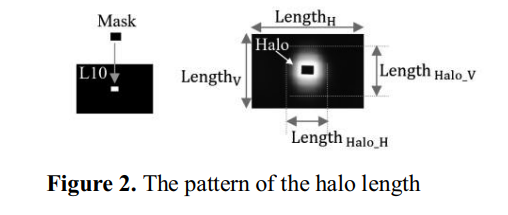After combining the human factors experiment, the halo is defined from the edge of the white display area to the area where the brightness of the halo is 0.002cd/m2 higher than that of the black background. Considering the size of the display, the halo length is defined as: halo length / length of the display. Halo lengths are used both vertically and horizontally.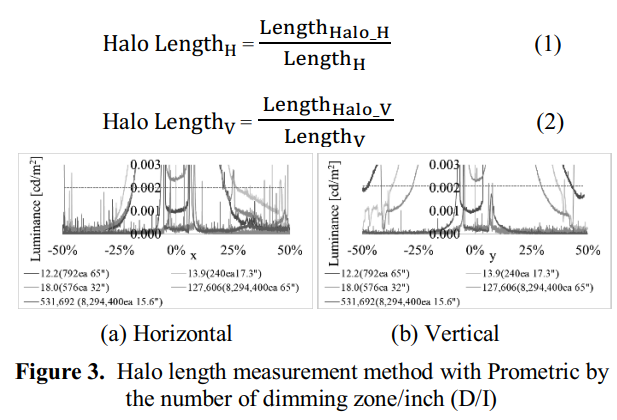According to the above method and measurement results, the larger the ratio of divisions/inch (D/I), the smaller the average length of the halo in the vertical and horizontal directions.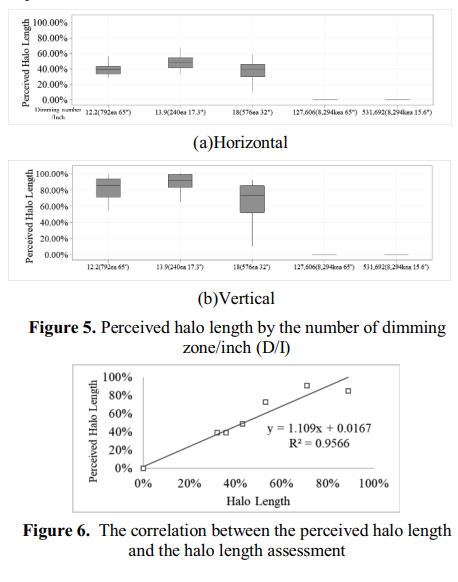To verify the significance of the definition of halo length, a subjective evaluation experiment was conducted on participants aged between 20 and 40 years. Participants were tasked with specifying where the halo ended horizontally and vertically. The observation distance is 0.65m, and the viewing angle is 30°, similar to Rtings conditions. The same 5 products were used. The graph below shows the perceived halo length and the correlation between the perceived and calculated halo length, which was significantly correlated (R2=0.9566). Therefore, it is believed that the halo length can be used as one of the indicators reflecting the actual contrast experience of the user.

Evaluation index 2: Angle halo Since the halo changes with the viewing angle, it is necessary to quantify the halo according to the viewing angle. Using the IDMS halo, the changes in viewing angle of the four products were observed. The results show that when the viewing angle of the four displays is larger and the halo is higher, the IDMS halo at the front view (0°) is the lowest. Therefore, the variance over viewing angle is defined as "angular halo", and it is evaluated.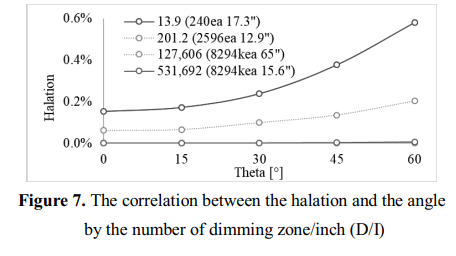Based on the IDMS halo definition, an image quality evaluation method is proposed to quantify the variance of halo over viewing angle, which is called angle halo.

For the experiment, a MiniLED LCD display with 240 divisions (17.3") and a self-illuminating display with 8294400 divisions (15.6") were selected (the number of divisions is equivalent to the number of pixels).

To compare the two monitors under the same conditions, the contrast ratio was set to 80000:1, while the halo length evaluation values (L10: 400cd/m2, full-screen black pattern: 0.0005cd/m2) were the same.

Halo value = 100% (center brightness of H10 - center brightness of full black point) / center brightness of L10 image

Calculate each halo value at 0-45 degrees, take the maximum value as the angle halo of this display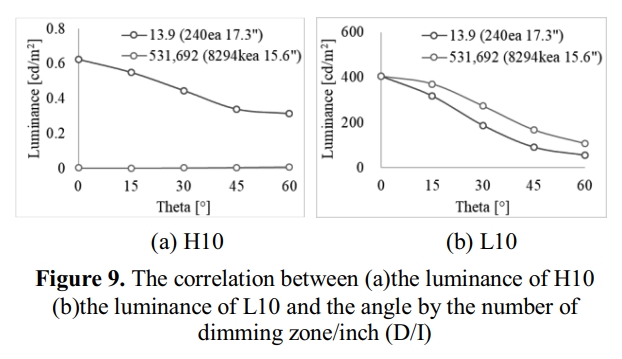The number of partitions per inch (D/I) is 531,692 (15.6") display, the halo value is 0.003%, and the front (0°) and side (45°) performance values are good. The D/I is 13.9 (17.3 ") display, 0.153% for the front (0°) and 0.377% for the side (45°). It was confirmed that the larger the number of divisions per inch (D/I), the smaller the intensity of the angular halo.

It is further verified by human factors experiments that the actual viewing angle halo perception of the human eye is very consistent with the angle halo value defined by the above formula.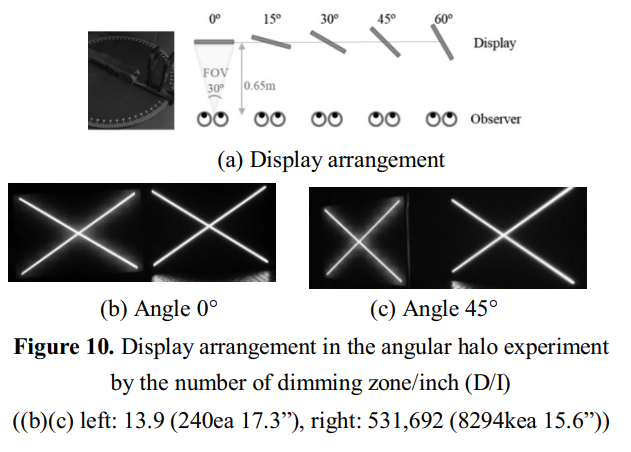Conclusion 1. There is a significant correlation between the perceived halo length and the calculated halo length (R2=0.9566), and the "halo length" can be evaluated in the following way: The halo is defined as the distance from the edge of the white display area to the brightness of the halo than the black background. In the area where the brightness is high by 0.002cd/m2, considering the size of the display, the halo length is defined as: halo length/display length. 2. In order to find the significant correlation between perceived angular halo and calculated angular halo (R2=0.8659), the angle halo can be evaluated as follows: halo value = 100% (central brightness of H10 - central brightness of full black field) / The central brightness of the L10 image is calculated at 0-45 degrees to calculate each halo value, and the maximum value is taken as the angle halo value of this display.

Source: MiniLED Industry Network

#### Keys:

LCF   LED display   full color LED display   MiniLED   small pitch LED display